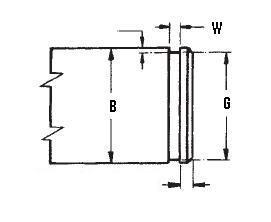### XAN-150

Display Units: inches |  metric
Request Quick Quote for this part.
 Ring Specs (D) Free Diameter: 1.408 +0.000 / -0.031 in. (t) Thickness: 0.050 +0.002 / -0.002 in. (b) Radial Wall: 0.109 +0.003 / -0.003 in. Groove Specs: (B) Application Diameter: 1.500 in. (G) Groove Diameter: 1.430 +0.004 / -0.004 in. (W) Groove Width: 0.056 +0.004 / -0.000 in. Groove Depth: 0.035 in. Other Specs Approximate Weight per 1000: (FG Max) Free Gap Max.: 0.312 in. (FG min) Free Gap Min.: 0.156 in. (Pg) Theoretical Thrust Load Capacity - Groove Yield:Notes: Yield Strength of Groove Material (Ys): 45,000 psi. Calculated using a safety factor (K) of 4Equation:Pg = [ B * d * Ys * pi ] / K    = [ (1.500 in.) * (0.035 in.) * (45,000 psi) * 3.14 ] / 4 1,854 lbs. (Pr) Theoretical Thrust Load Capacity - Ring Shear:Notes: Ring Material: Carbon Spring Steel (SAE 1060-1095). Shear Strength of Ring Material (Ss): 153,000 psi. Calculated using a safety factor (K) of 3Equation:Pr = [ B * t * Ss * pi ] / K    = [ (1.500 in.) * (0.050 in.) * (153,000 psi) * 3.14 ] / 3 12,010 lbs. Industry Equivalent Part Number(s):XAN-150